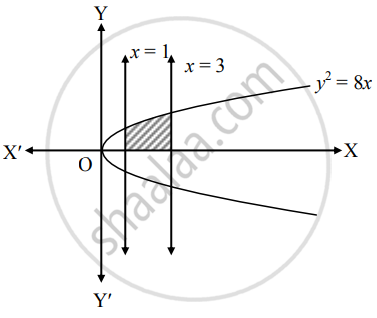# Find the area of the region bounded by the curve y2 = 8x, the X−axis and the given lines x = 1, x = 3, y ≥ 0 - Mathematics and Statistics

Sum

Find the area of the region bounded by the curve y2 = 8x, the X−axis and the given lines x = 1, x = 3, y ≥ 0

#### Solution

Let A be the required area.

Consider the equation y2 = 8x.∴ A = int_1^3 y  "d"x

= int_1^3 sqrt(8x)  "d"x

= 2sqrt(2)[(x^(3/2))/(3/2)]_1^3

= (4sqrt(2))/3 [(3)^(3/2) - (1)^(3/2)]

= (4sqrt(2))/3 (3sqrt(3) - 1) sq.units

Concept: Area Bounded by the Curve, Axis and Line
Is there an error in this question or solution?